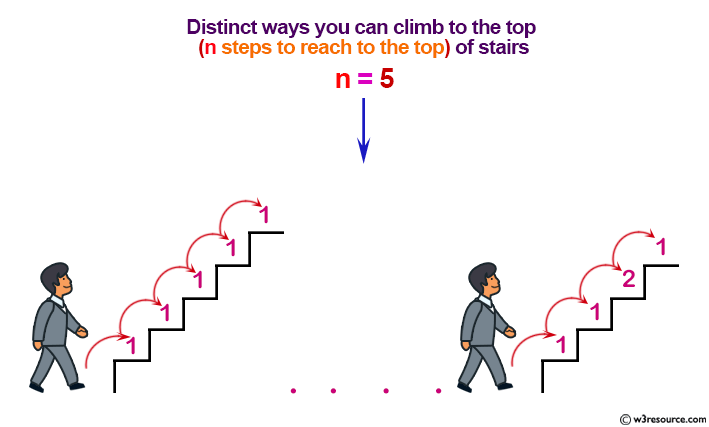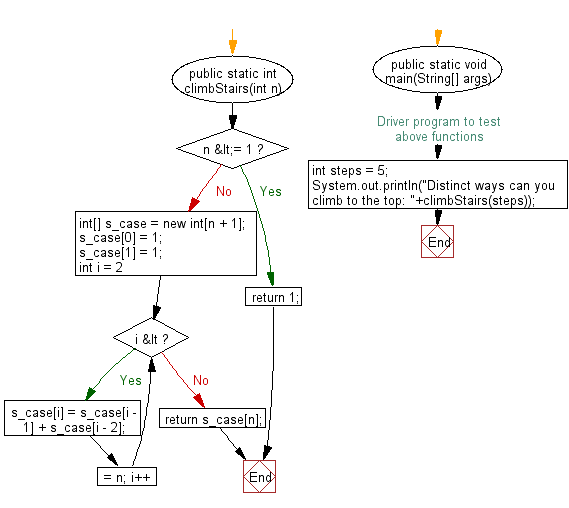﻿ Java exercises: Find the distinct ways you can climb to the top - w3resource# Java Exercises: Find the distinct ways you can climb to the top

## Java Basic: Exercise-134 with Solution

Write a Java program to find the distinct ways you can climb to the top (n steps to reach to the top) of stairs. Each time you can either climb 1 or 2 steps.

Example: n = 5 a) 1+1+1+1+1 = 5 b) 1+1+1+2 = 5 c) 1+2+2 = 5 d) 2+2+1 = 5 e) 2+1+1+1 = 5 f) 2+1+2 = 5 g) 1+2+1+1 = 5 h) 1+1+2+1 = 5

Pictorial Presentation:Sample Solution:

Java Code:

``````class Solution {
public static int climbStairs(int n) {
if (n <= 1) {
return 1;
}
int[] s_case = new int[n + 1];
s_case = 1;
s_case = 1;
for (int i = 2; i <= n; i++) {
s_case[i] = s_case[i - 1] + s_case[i - 2];
}
return s_case[n];
}
/* Driver program to test above functions */
public static void main(String[] args) {
int steps = 5;
System.out.println("Distinct ways can you climb to the top: "+climbStairs(steps));
}
}
```
```

Sample Output:

```Distinct ways can you climb to the top: 8
```

Flowchart:Java Code Editor:

Company:

What is the difficulty level of this exercise?

﻿

## Java: Tips of the Day

initial:

Returns all the elements of an array except the last one. Use Arrays.copyOfRange() to return all except the last one

```public static <T> T[] initial(T[] elements) {
return Arrays.copyOfRange(elements, 0, elements.length - 1);
}
```

Ref: https://bit.ly/3zEKw9u